# Summary of Chapter 5, SVM 第五节总结，向量机

What is SVM? Why is it so frequently mentioned? What makes it so powerful? Let’s take a look. 什么是支持向量机？为什么这么火？怎么就那么强大？让我们一探究竟。

In this chapter summary, I will show how SVM works. Linear algebra behind the model is not the focus. 在这节总结中，我将展示向量机的简单原理。模型中的线性代数不被讨论。故而篇幅略短

Table of Content. 内容提要

1. Linear SVM Classification 线性向量机分类
1. Hard Margin
2. Soft Margin
2. Nonlinear SVM Classification 非线性向量机分类
3. SVM Regression 向量机回归

Support Vector Machine (SVM) is a very powerful and versatile Machine Learning model. It can be applied to many types of problems, classification, regression, etc. 向量机很好很强大。可以用到很多方面。

IMPORTANT!!! SVM is especially useful for classification of complex but small- or medium-sized datasets.

### Linear SVM Classification 线性向量机分类问题

We can think the classification problem is to separate datasets into different groups with minimum uncertainty, while different models give different definitions of “uncertainty”.

Many classification models consider most of the data points in the datasets. Recall that in Logistic Regression, the score function comes from the mean of log-likelihood. In Decision Tree, at each leaf, all data points are used to calculate the score to produce decision rules.

On the other hand, SVM does not consider all data points, not even the majority of data points. The model is decided by only a few data points out of the whole datasets. Take a look at data points below, it is obvious that only those points near the separation border affect the final model.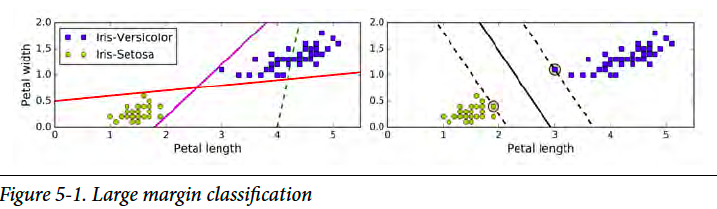#### Hard Margin （硬间隔）

Let’s assume the datasets are linearly separatable. That is to say, there exists a hyperplane that can completely and cleanly separate the datasets into different classes. In a 2-D 2-classes case, there exists a line separating the datasets. So here is the question, as there are more than one possible lines, which one gives minimum uncertainty? To answer this question, let’s first define a margin variable as the distance from the hyperplane to the nearest training data point of any class. In SVM, the larger this distance is, the less uncertainty there is. The score function is to maximize this distance.

Here is the objective function for hard margin linear SVM. (It is Ok if you do not understand. The formulation is presented here as I want to show the comparison of hard margin and soft margin.)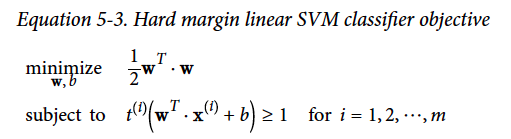#### Soft Margin （软间隔）

What if the datasets are not separatable? Then we need soft margin. We use a parameter C to adjust the “softness”. Another advantage of soft margin is insensitive to outliers. The “softer” the model is, the model is more tolerable to margin violations.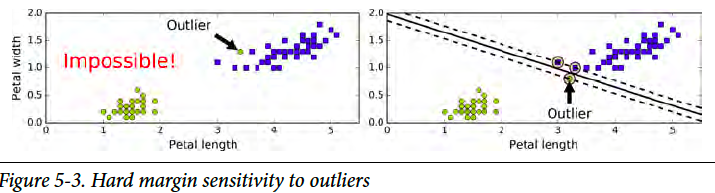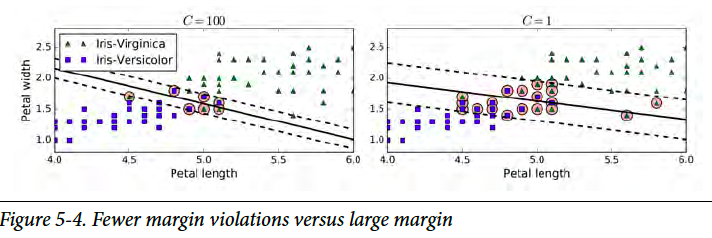Here is the objective function for hard margin linear SVM. (It is Ok if you do not understand. The formulation is presented here as I want to show the comparison of hard margin and soft margin.) 下面是软边际的目标方程。（不懂也没关系，这个方程只是为了展示硬软间隔的区别。想知更多，请查专业书籍）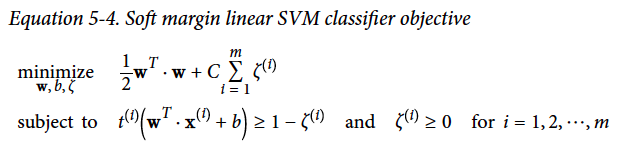### Nonlinear SVM Classification 非线性向量机分类

It is ideal if datasets can be linearly separated by a straight line or a hyperplane. However, life is hard. We have to handle non-linear cases. The approach is to transform response variables (X) so that the problem can be fitted to linear SVM. The original datasets (left) cannot be separated by a line. However, after transforming X into higher-dimension datasets like the figure on the right.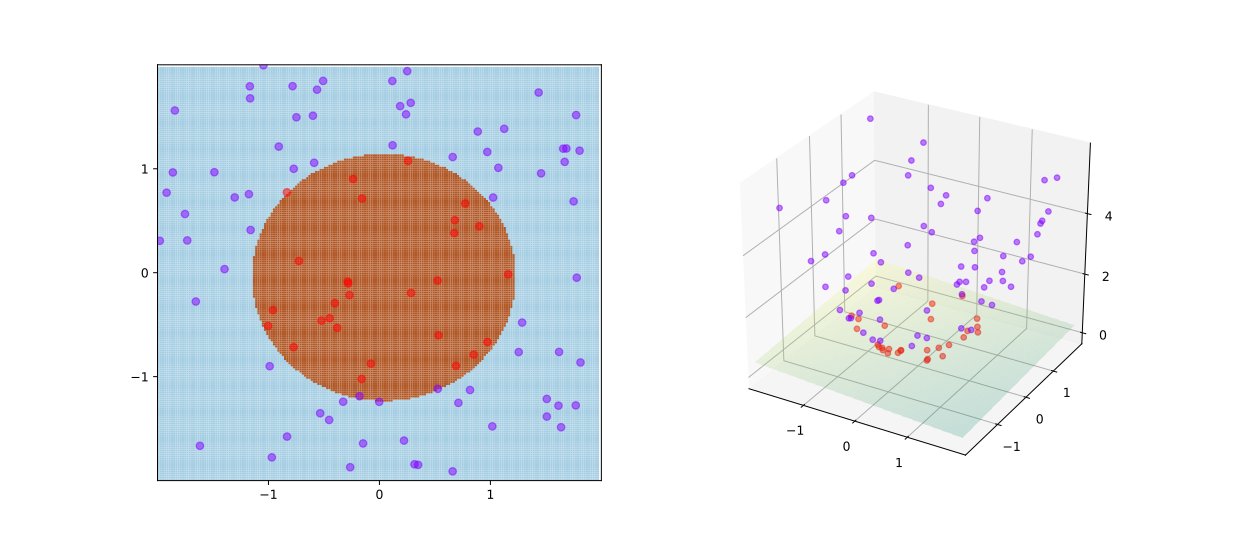To implement the transformation, scikit-learn apply a technique called kernel trick. Common kernels include Polynomial Kernel and Gaussian RBF Kernel.

from sklearn.svm import SVC
SVC(kernel="poly", degree=3, coef0=1, C=5) # Polynomial Kernel
SVC(kernel="rbf", gamma=5, C=0.001) # Gaussian RBF Kernel


### SVM Regression 向量机回归

For a regression problem, we usually picture the data points are scattered around the fitted line. Comparably, in a classification problem, the data points are scattered away from the borderline. So do you notice the similarity? Yes, by reversing the idea of SVM classifier, we obtain the SVM regressor. Instead of trying to exclude as many data points as possible in the border band in SVM classifier, we try to include as many data points in the regression line band in SVM regressor.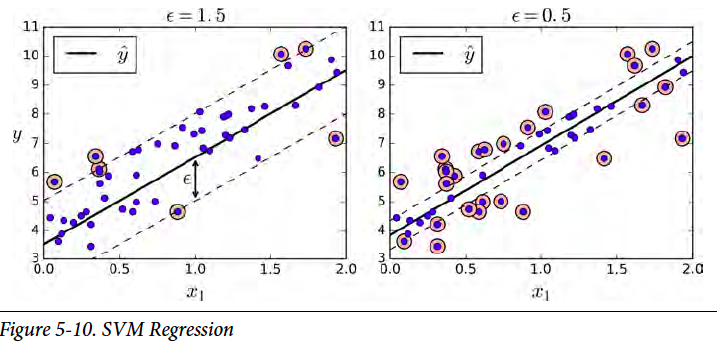This article is part of a series of summaries on the book Hands-On Machine Learning with Scikit-Learn and TensorFlow. The summaries are meant to explain machine learning concepts and ideas, instead of covering the maths and models.

Written on April 2, 2018